# Nickzom Calculator Calculates Range and Maximum Range of a Projectile in Physics

To get the answer for the range of a projectile, there are three essential parameters to be given which are:

• Acceleration due to Gravity
• Initial Velocity
• Angle of Projection

Where as to get the answer for maximum range of a projectile requires two parameters to be given which are:

• Acceleration due to Gravity
• Initial Velocity

Let’s first, proceed to the home page of the app. Select Physics and click on Open Section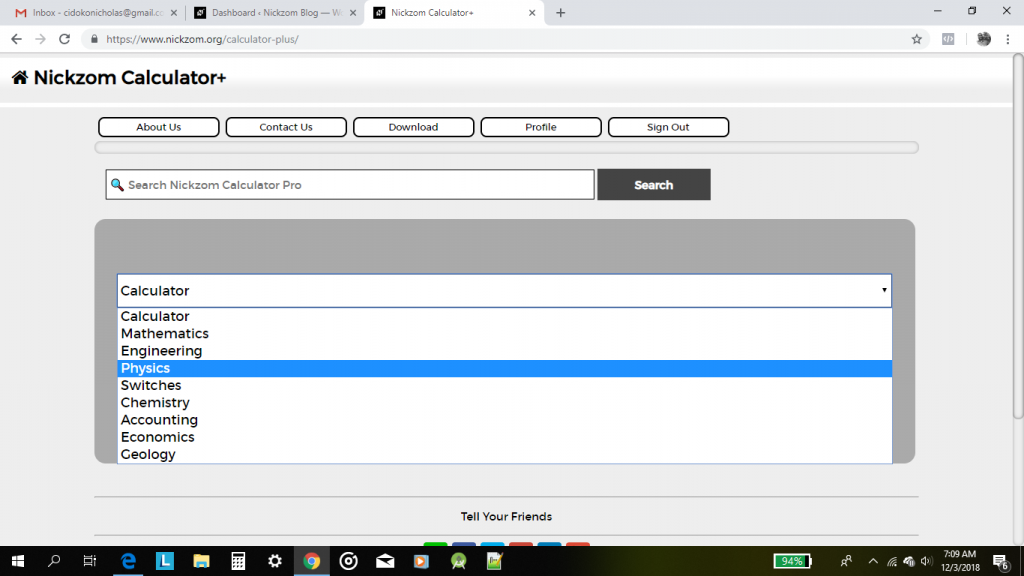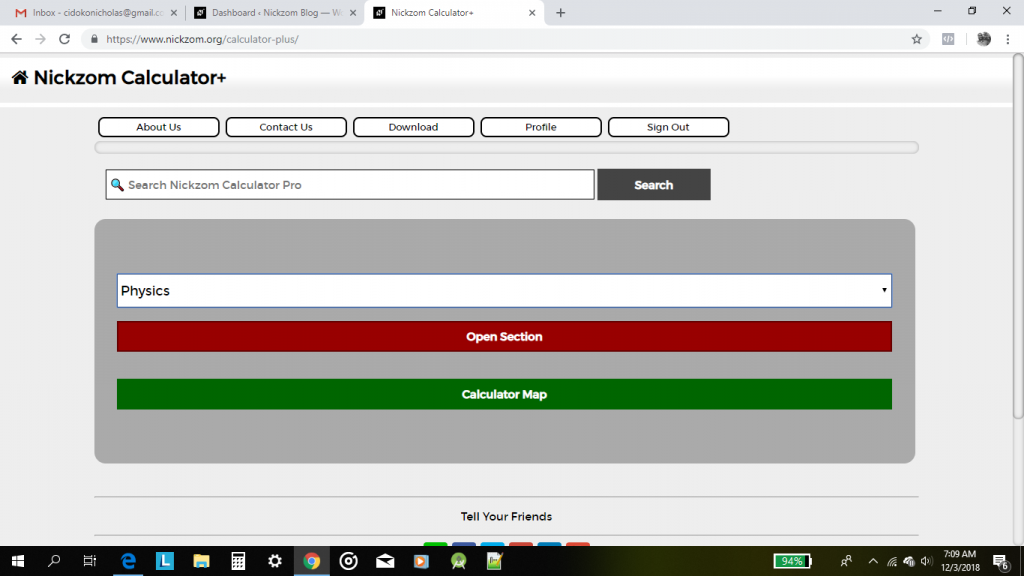Then proceed to click on ProjectileLet’s first get an answer for a simple projectile problem using Nickzom Calculator.

A motorcyclist has set up a stunt with a ramp at the edge of a gorge. The ramp is inclined at 53.1° from the horizontal plane. She plans to take off from the ramp at a velocity of 28.0 m/s. At that velocity, what will be her horizontal range at an acceleration due to gravity of 9.81m/s2?

Now, we would click on Range in the Projectile Page.This page below comes up.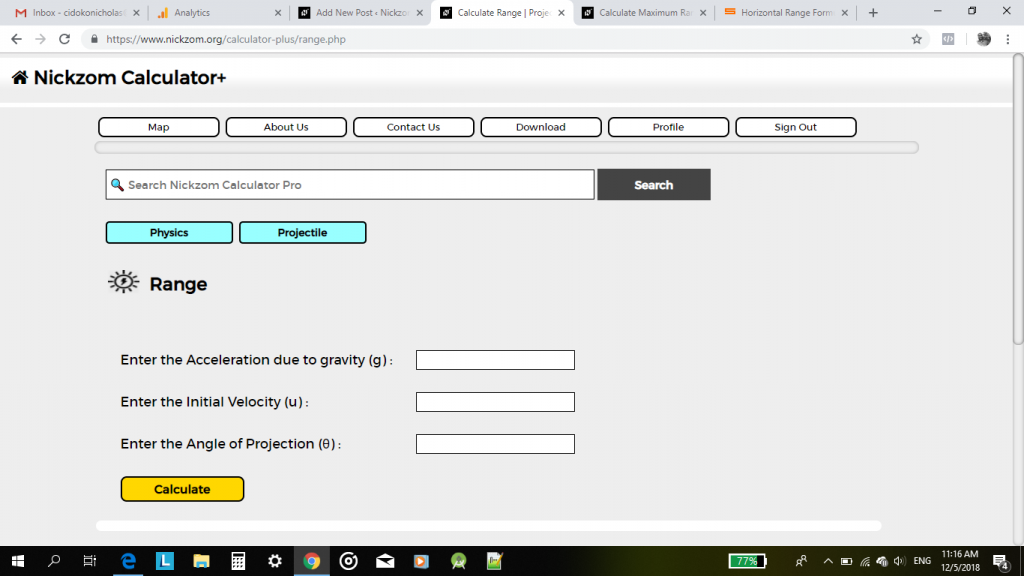We already know the values of the parameters now, let us state them out and input them accurately and appropriately into Nickzom Calculator.

Acceleration due to Gravity: 9.81

Initial Velocity: 28

Angle of Projection: 53.1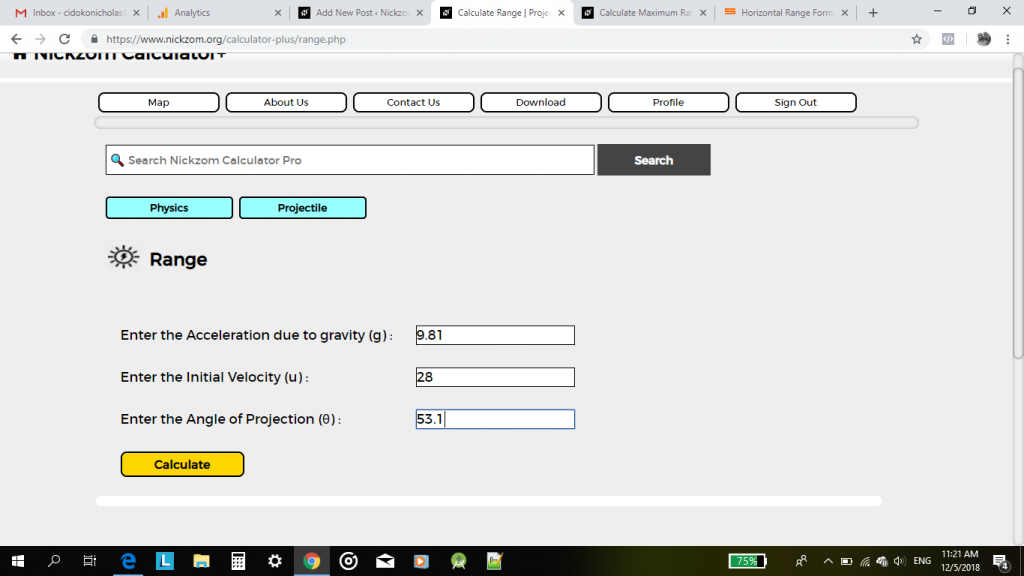Now, all that is left is to click on Calculate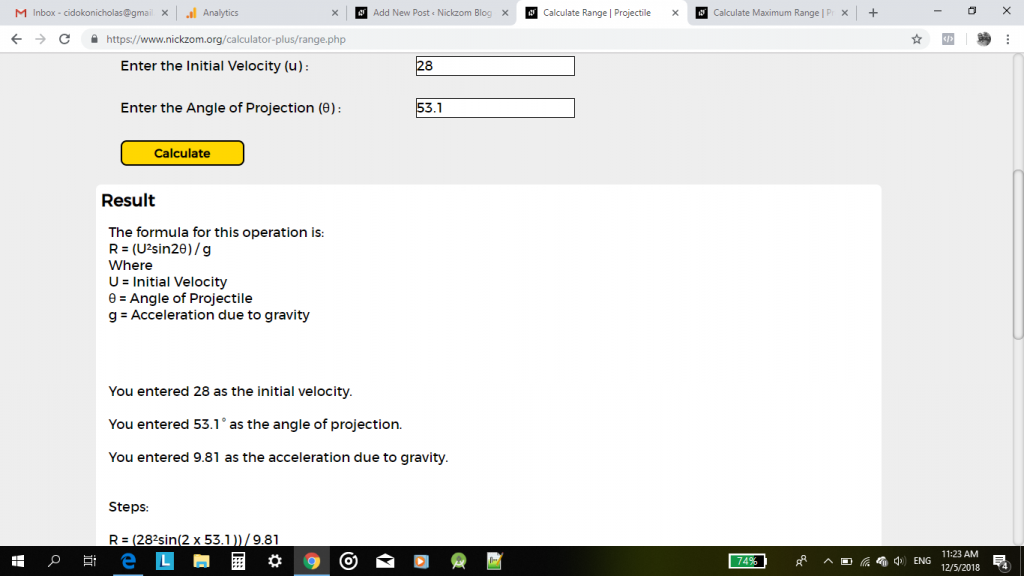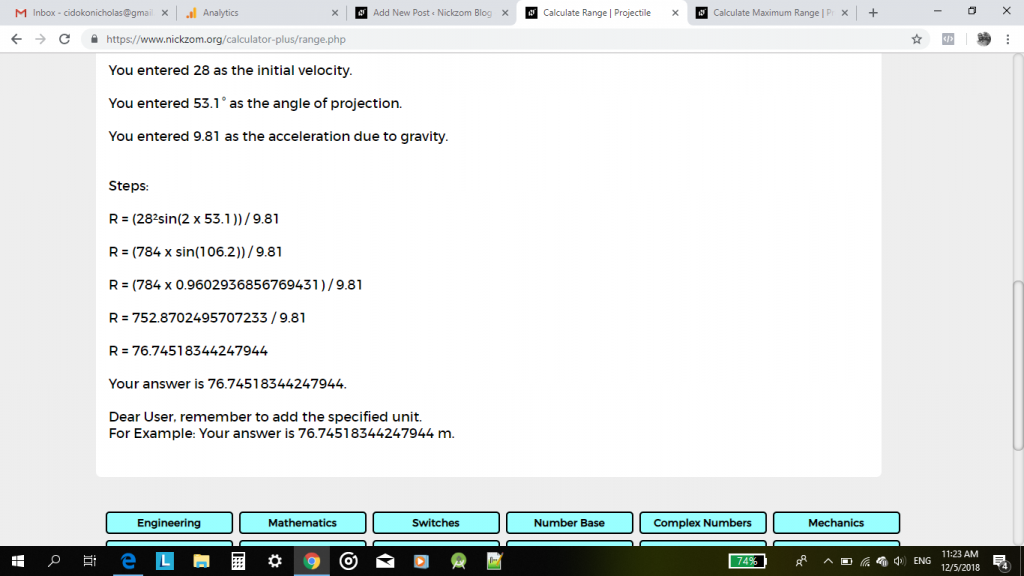You can clearly see from the screenshot above that the range is 76.75 m.

Great, let’s now use Nickzom Calculator to get answers for maximum range of a projectile.

What is the maximum range of a projectile with an initial velocity of 30 m/s and and acceleration due to gravity of 10 m/s2?

Navigate back to the Projectile page and click on Maximum RangeA page with the two parameters for you to enter is displayed.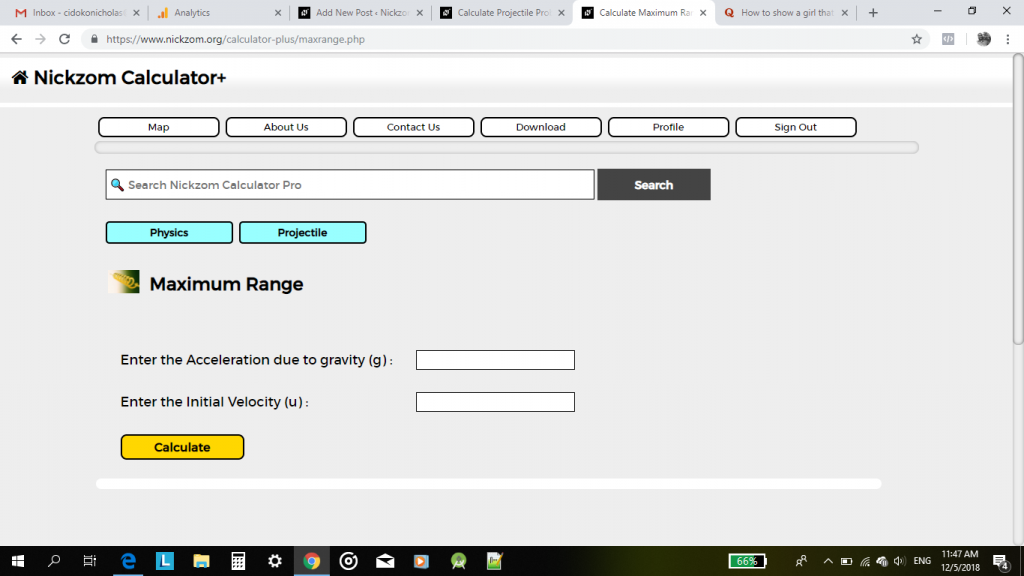Identifying the parameters and entering the values

Acceleration due to Gravity: 10

Initial Velocity: 30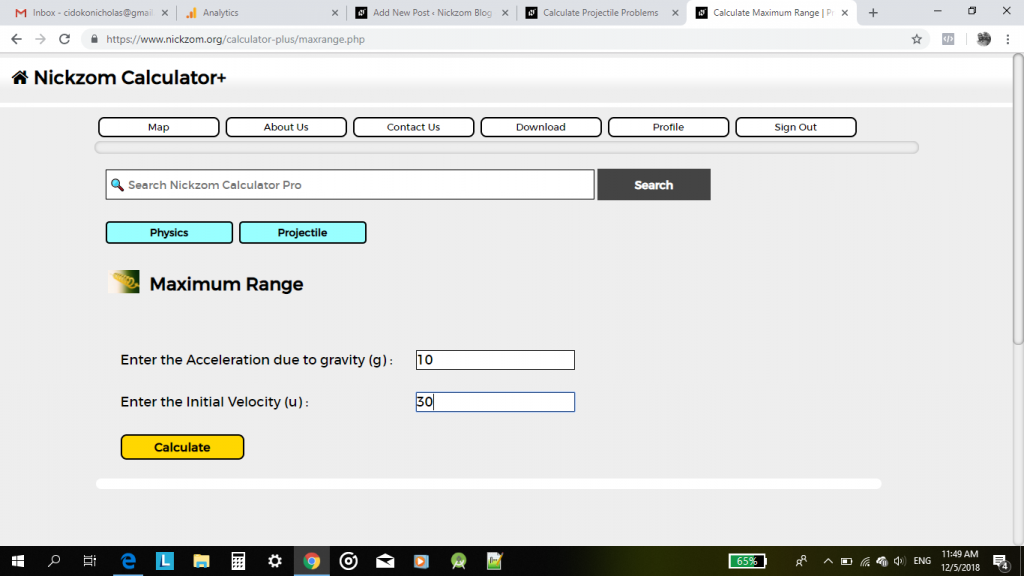Finally, click on Calculate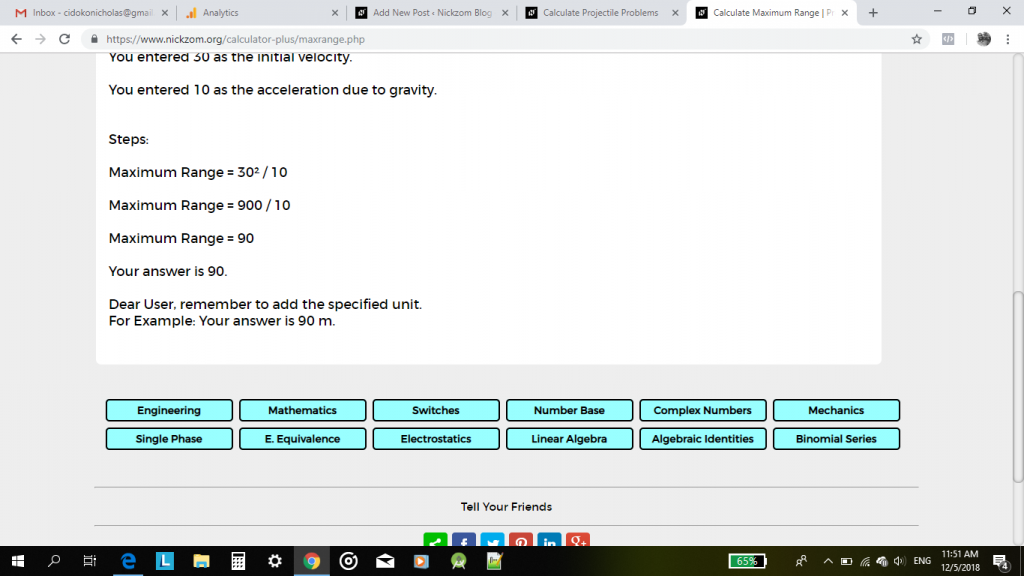As we can see from the screenshot above, the maximum range is 90 m.

You can access Nickzom Calculator (The Calculator Encyclopedia) via any of these channels:

## One thought on “Nickzom Calculator Calculates Range and Maximum Range of a Projectile in Physics”

1.cresent moon cafe says:

Very great post. I simply stumbled upon your weblog and wished to mention that I’ve truly enjoyed browsing your weblog posts.
After all I’ll be subscribing for your feed and I’m hoping you write again soon!